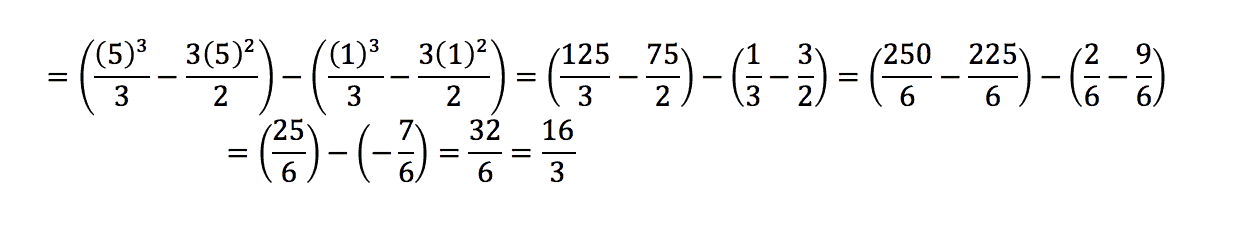# PCAT Quantitative Reasoning – Area Under the Curve Between x = 1 and x = 5

Find the area under the curve for the function f(x) = x2 – 3x – 1 between x = 1 and x = 5.

A. 8/3
B. 3
C. 16/3
D. 8

##### Click for Explanation

C is correct. This problem is asking for the definite integral of the function given. First, we will need to find the antiderivative. Remember to add the integration constant C:Next, let’s plug in x = 5 and then subtract the result when x = 1:#### Submit a Comment

This site uses Akismet to reduce spam. Learn how your comment data is processed.